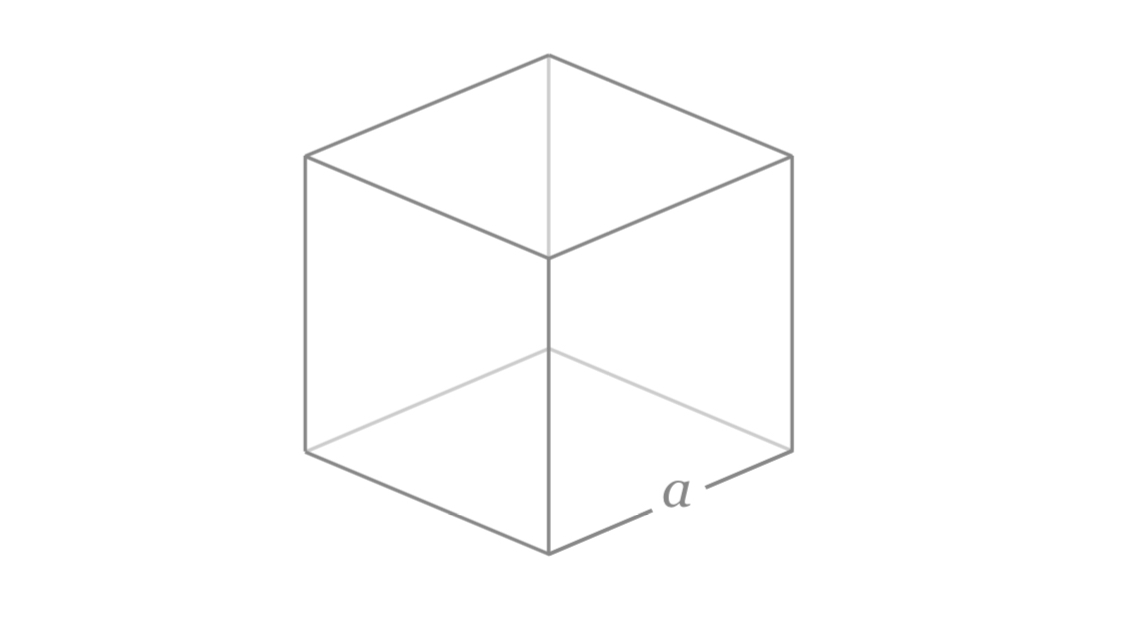# The side length cube is 3.78 times 10^3 ft. What is the volume of the cube?

The side length cube is 3.78 times 10^3 ft. What is the volume of the cube?

Answer 1

5.4 × 10^10\ "ft"^3

#### Explanation:Volume of cube of side length $\text{a}$ is ${\text{a}}^{3}$

${\text{V" = (3.78 × 10^3\ "ft")^3 ≈ 5.4 × 10^10\ "ft}}^{3}$

#### Earn Coin

Coins can be redeemed for fabulous gifts.

Similar Homework Help Questions

• ### Length of of a side of a cube, if the volume is 1000 cubic meters

Length of of a side of a cube, if the volume is 1000 cubic meters?

• ### The volume of a cube is given by V = s3, where s is the length of a side

The volume of a cube is given by V = s3, where s is the length of a side. Find the length of a side of a cube if the volume is 500 cm3. Round answer to three decimal places